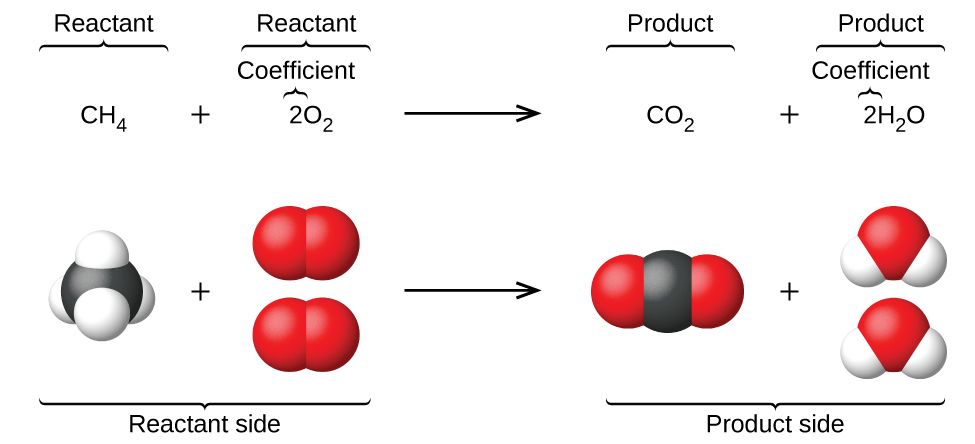# Word Equation Study Guide

Introduction

Cookbooks with a bunch of delicious recipes are super useful to whip up a dish that is equally delicious each time it is made. The same idea can be applied to chemical reactions! How can we note down chemical reactions so that you can go through them later?

## WHAT ARE CHEMICAL EQUATIONS?

You might not notice, but chemical equations are happening all around you.

• Plants in the presence of sunlight drive their photosynthetic process to produce energy.
• Vehicles burn gasoline and fuel to power their engines.
• You would notice batteries using electrochemical reactions to power devices by producing energy.
• You would not notice, but several chemical reactions are happening within the human body, too, particularly when it concerns food digestion.

## WHAT ARE CHEMICAL WORD EQUATIONS?

A chemist can’t remember every equation and every reaction occurring accurately. This is why they keep track of equations by noting them down. In every chemical reaction, one or more substances termed reactants are converted to one or more new substances termed as products. The general equation for this would be

Reactants → Products

You must not use an equal sign while representing a chemical reaction.Source

## CHEMICAL EQUATION TO WORD EQUATION

• In chemistry, a word equation represents a chemical reaction in words rather than using chemical formulas.
• A word equation usually states the reactants and the products and the direction in which the chemical equation is proceeding.
• There are some key factors that you should keep in mind while writing word equations for chemical reactions.
1. The words ‘and’ or ‘plus’ mean one chemical and another are both reactants or products.

2. The phrase ‘is reacted with,’ states that those chemicals are reactants.

3. Using the words ‘forms’, ‘yields,’ ‘makes’ means the chemicals are products.

4. Reactants would always be mentioned on the left side while the products are on the right side.

## EXAMPLES OF WORD CHEMICAL EQUATIONS

Let us take the chemical reaction

2H2(g) + O2(g) → 2H20(g)

This, in word equation, would be expressed as

Hydrogen gas + Oxygen gas → Steam

• In most word equations, you would not use numbers or symbols; however, in some reactions, like the transition metals, it is necessary to indicate the oxidation state of the reactant.
• Examples would be the reaction between oxygen and copper to form copper oxide.
• The number of oxygen and copper atoms involved completely depends on whether copper (I) or copper (II) is involved.

Copper + Oxygen → Copper (II) oxide

Another one would be

2Cu + O2→ 2CuO

Here, the chemical equation is completely different, and copper (I) has been used.

# SUMMARY

• A word equation is an expression of a chemical reaction.
• Word equations helps us understand the order of events of chemical equations.

## FAQs

1. What are the three common components of a word equation?

The three common components of a word equation are reactants, products, and the direction of the reaction.

2. What does a chemical equation show that a word equation does not?

The chemical equations showcase the chemical formulas that word equations do not.

We hope you enjoyed studying this lesson and learned something cool about Word Equations! Join our Discord community to get any questions you may have answered and to engage with other students just like you! Don’t forget to download our App to experience our fun VR classrooms – we promise, it makes studying much more fun!😎

## REFERENCE

1. Word Equations: https://chem.libretexts.org/Bookshelves/Introductory_Chemistry/Introductory_Chemistry_(CK-12)/11%3A_Chemical_Reactions/11.01%3A_Word_Equations. Accessed 8th March 2022.
2. Definition of Word Equation: https://www.thoughtco.com/definition-of-word-equation-605801. Accessed 8th March 2022.
]]>